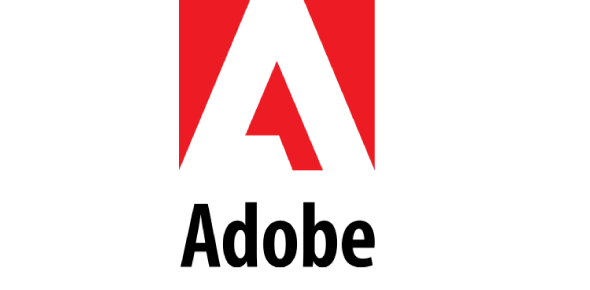# OCP C: Indesign CS2 Quiz - Chapters 7 & 8

40 Questions | Attempts: 313
ShareSettingsYou will have 30 minutes to complete this 40-question written test covering InDesign chapters 7 & 8. Once completed, print your certificate and ask for the practical portion (if not already completed). Good Luck!

• 1.
In Photoshop, the Pen Tool is used mostly to draw vector graphice.
• A.

T

• B.

F

• 2.
You can apply fills and strokes to closed paths.
• A.

T

• B.

F

• 3.
It is possible that the graphics in the figure above was creaqted with the Ellipse Tool.
• A.

T

• B.

F

• 4.
Inline frames flow with text.
• A.

T

• B.

F

• 5.
In Photoshop, Grayscale is a color mode.
• A.

T

• B.

F

• 6.
If an object is opaque, it can be translucent but not transparent.
• A.

T

• B.

F

• 7.
Blending modes allow you to create different transparency and color effects where two or more objects overlap.
• A.

T

• B.

F

• 8.
Blending modes are used only to create dramatic special effects.
• A.

T

• B.

F

• 9.
Blending modes can be applied to text.
• A.

T

• B.

F

• 10.
Multiply is a good solution for removing the white background of a placed graphic.
• A.

T

• B.

F

• 11.
Teh letter U is and example of _________.
• A.

An open path

• B.

A closed path

• C.

A cap

• D.

None of the above

• 12.
To create a path around a tomato, you'd need to create ________.
• A.

An open path

• B.

A closed path

• C.

A line

• D.

None of the above

• 13.
Teh letter D is an example of _______.
• A.

A join

• B.

A closed path

• C.

An open path

• D.

None of the above

• 14.
A straight line is an example of ____________.
• A.

An open path

• B.

A closed path

• C.

None of the above

• D.

Both A and B

• 15.
An open path is a path whise end points are ________.
• A.

Straight

• B.

Not connected

• C.

Pointed

• D.

Connected

• 16.
In the figure above, what part of the path is identified by the letter "E."
• A.

End point

• B.

Path segment

• C.

Corner point

• D.

Smooth point

• 17.
In the figure, above what part of the path is identified by the letter "C."
• A.

End point

• B.

Path segment

• C.

Corner Point

• D.

Smooth point

• 18.
In the figure, above what part of the path is identified by the letter "B."
• A.

End point

• B.

Path segment

• C.

Corner Point

• D.

Smooth point

• 19.
Hen the Pen Tool is positioned over an anchor point, it automatically changes to this tool.
• A.

Selection tool

• B.

Direct selection tool

• C.

Both A and B

• D.

None of the above

• 20.
When you click an anchor point with the Delete Anchor Tool ______.
• A.

The anchor point is deleted

• B.

The anchor point is deleted and any line segments attached to the point are also deleted

• C.

The entire image is deleted

• D.

• 21.
When the Pen Tool sis positioned over a line segment, it automatically chages this tool.
• A.

Selection tool

• B.

Direct sleetion tool

• C.

Both A and B

• D.

None of the above

• 22.
Of the three types of joins, which produces square corners?
• A.

Miter

• B.

Round

• C.

Bevel

• D.

All of the above

• 23.
Pressing [Alt] (Win) or [Option] (Mac) when drawing a circle allows you to do what?
• A.

Delete the background image

• B.

Draw the circle from its center

• C.

Draw a rectangle

• D.

Constrain the circle as a perfect circle

• 24.
Pressing and holding [Shift] when drawing a circle allows you to do what?
• A.

Constrain the circle as a perfect circle

• B.

Draw the circle from its center

• C.

Deleted the circle

• D.

Draw a rectangle

• 25.
The figure above is _________.
• A.

An open path

• B.

A closed path

• C.

A path segment

• D.

An end point

## Related TopicsBack to top
×

Wait!
Here's an interesting quiz for you.